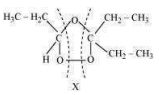Question 13.7:-

Propanal and pentane-3-one are the ozonolysis products of an alkene? What is the structural formula of the alkene?

As per the given information, propanal and pentan-3-one are the ozonolysis products of an alkene. Let the given alkene be ‘A’. Writing the reverse of the ozonolysis reaction, we
get:

${H}_{3}C-C{H}_{2}-CHO+C{H}_{2}-\underset{\underset{O}{\parallel }}{C}-C{H}_{2}-C{H}_{3}$



The products are obtained on the cleavage of ozonide ‘X’. Hence, ‘X’ contains both products in the cyclic form. The possible structure of ozonide can be represented as:Now, ‘X’ is an addition product of alkene ‘A’ with ozone. Therefore, the possible structure of alkene ‘A’ is:

${H}_{3}C-C{H}_{2}-CH=C-C{H}_{2}-C{H}_{3}$